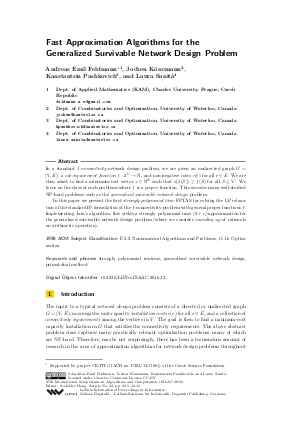Document# Fast Approximation Algorithms for the Generalized Survivable Network Design Problem

### Authors Andreas Emil Feldmann, Jochen Könemann, Kanstantsin Pashkovich, Laura Sanità## File

LIPIcs.ISAAC.2016.33.pdf
• Filesize: 0.5 MB
• 12 pages

## Cite As

Andreas Emil Feldmann, Jochen Könemann, Kanstantsin Pashkovich, and Laura Sanità. Fast Approximation Algorithms for the Generalized Survivable Network Design Problem. In 27th International Symposium on Algorithms and Computation (ISAAC 2016). Leibniz International Proceedings in Informatics (LIPIcs), Volume 64, pp. 33:1-33:12, Schloss Dagstuhl - Leibniz-Zentrum für Informatik (2016)
https://doi.org/10.4230/LIPIcs.ISAAC.2016.33

## Abstract

In a standard f-connectivity network design problem, we are given an undirected graph G = (V, E), a cut-requirement function f : 2^V to N, and non-negative costs c(e) for all e in E. We are then asked to find a minimum-cost vector x in N^E such that x(delta(S)) geq f (S) for all S subseteq V. We focus on the class of such problems where f is a proper function. This encodes many well-studied NP-hard problems such as the generalized survivable network design problem. In this paper we present the first strongly polynomial time FPTAS for solving the LP relaxation of the standard IP formulation of the f-connectivity problem with general proper functions f. Implementing Jain’s algorithm, this yields a strongly polynomial time (2 + epsilon)-approximation for the generalized survivable network design problem (where we consider rounding up of rationals an arithmetic operation).
##### Keywords
• strongly polynomial runtime
• generalized survivable network design
• primal-dual method

## Metrics

• Access Statistics
• Total Accesses (updated on a weekly basis)
0

## References

1. Sanjeev Arora, Elad Hazan, and Satyen Kale. The multiplicative weights update method: a meta-algorithm and applications. Theory of Computing, 8(1):121-164, 2012.2. Daniel Bienstock. Potential function methods for approximately solving linear programming problems: theory and practice, volume 53. Springer Science &Business Media, 2006.3. Daniel Bienstock and Garud Iyengar. Solving fractional packing problems in o ast (1/ε) iterations. In Proc. of the 36th Annual ACM Symp. on Theory of Computing, pages 146-155. ACM, 2004.4. Khaled Elbassioni, Kurt Mehlhorn, and Fahimeh Ramezani. Towards more practical linear programming-based techniques for algorithmic mechanism design. SAGT, pages 98-109, 2015.5. Lisa Fleischer. A fast approximation scheme for fractional covering problems with variable upper bounds. In Proceedings of the fifteenth annual ACM-SIAM symposium on Discrete algorithms, pages 1001-1010. Society for Industrial and Applied Mathematics, 2004.6. Lisa K. Fleischer. Approximating fractional multicommodity flow independent of the number of commodities. SIAM Journal on Discrete Mathematics, 13(4):505-520, 2000.7. Harold N. Gabow, Michel X. Goemans, and David P. Williamson. An efficient approximation algorithm for the survivable network design problem. Mathematical Programming, 82(1-2, Ser. B):13-40, 1998. Networks and matroids; Sequencing and scheduling.8. Naveen Garg and Rohit Khandekar. Fast approximation algorithms for fractional steiner forest and related problems. In Foundations of Computer Science, 2002. Proceedings. The 43rd Annual IEEE Symposium on, pages 500-509. IEEE, 2002.9. Naveen Garg and Rohit Khandekar. Fractional covering with upper bounds on the variables: Solving lps with negative entries. In Algorithms-ESA 2004, pages 371-382. Springer, 2004.10. Naveen Garg and Jochen Könemann. Faster and simpler algorithms for multicommodity flow and other fractional packing problems. SIAM J. Comput., 37(2):630-652, 2007. URL: http://dx.doi.org/10.1137/S0097539704446232.
11. M. X. Goemans, D. B. Shmoys, A. V. Goldberg, É. Tardos, S. Plotkin, and D. P. Williamson. Improved approximation algorithms for network design problems. In Proc. of the 5th Annual ACM-SIAM Symp. on Discrete Algorithms (SODA'94), pages 223-232. ACM, 1994.12. Michel X Goemans and David P Williamson. A general approximation technique for constrained forest problems. SIAM Journal on Computing, 24(2):296-317, 1995.13. R. E. Gomory and T. C. Hu. Multi-terminal network flows. J. Soc. Indust. Appl. Math., 9:551-570, 1961.14. Michael D Grigoriadis and Leonid G Khachiyan. Fast approximation schemes for convex programs with many blocks and coupling constraints. SIAM Journal on Optimization, 4(1):86-107, 1994.15. Michael D Grigoriadis and Leonid G Khachiyan. Approximate minimum-cost multicommodity flows in ̃ o(ε - 2 knm) time. Mathematical Programming, 75(3):477-482, 1996.16. Martin Grötschel, László Lovász, and Alexander Schrijver. Geometric algorithms and combinatorial optimization, volume 2 of Algorithms and Combinatorics: Study and Research Texts. Springer-Verlag, Berlin, 1988. URL: http://dx.doi.org/10.1007/978-3-642-97881-4.
17. Anupam Gupta and Jochen Könemann. Approximation algorithms for network design: A survey. Surveys in Operations Research and Management Science, 16(1):3-20, 2011.18. Dorit S Hochbaum. Approximation algorithms for NP-hard problems. PWS Publishing Co., 1996.19. Kamal Jain. A factor 2 approximation algorithm for the generalized Steiner network problem. Combinatorica, 21(1):39-60, 2001.20. Michael Luby and Noam Nisan. A parallel approximation algorithm for positive linear programming. In Proceedings of the twenty-fifth annual ACM symposium on Theory of computing, pages 448-457. ACM, 1993.21. Farhad Shahrokhi and David W Matula. The maximum concurrent flow problem. Journal of the ACM (JACM), 37(2):318-334, 1990.22. Eva Tardos. A strongly polynomial algorithm to solve combinatorial linear programs. Operations Research, 34(2):250-256, 1986.23. David P. Williamson and David B. Shmoys. The design of approximation algorithms. Cambridge University Press, Cambridge, 2011. URL: http://dx.doi.org/10.1017/CBO9780511921735.
24. Neal E Young. Randomized rounding without solving the linear program. In SODA, volume 95, pages 170-178, 1995.25. Neal E Young. Sequential and parallel algorithms for mixed packing and covering. In Foundations of Computer Science, 2001. Proceedings. 42nd IEEE Symposium on, pages 538-546. IEEE, 2001.X

Feedback for Dagstuhl Publishing